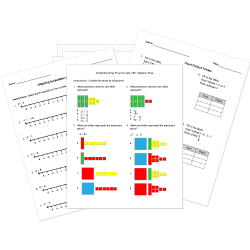Looking for Algebra worksheets?
Check out our pre-made Algebra worksheets!
 Tweet##### Browse Questions
• Arts (1039)
• English Language Arts (5423)
• English as a Second Language ESL (3251)
• Health and Medicine (901)
• Life Skills (399)
• Math (2919)

• ### Systems of Equations

• #### Statistics and Probability Concepts

• Physical Education (845)
• Science (7678)
• Social Studies (3990)
• Study Skills and Strategies (159)
• Technology (365)
• Vocational Education (817)

You can create printable tests and worksheets from these Grade 9 Sequences and Series questions! Select one or more questions using the checkboxes above each question. Then click the add selected questions to a test button before moving to another page.

Previous Next
Grade 9 Sequences and Series CCSS: HSF-BF.A.2
Given the explicit form of the arithmetic sequence $a_n = -2 + 7n$, which of the following is the recursive formula for the same sequence?
1. $a_1 = 5; \ \ a_n = a_{n-1} + 7, \ n>1$
2. $a_1 = 5; \ \ a_n = a_{n-1} - 2, \ n>1$
3. $a_1 = -2; \ \ a_n = a_{n-1} +7, \ n>1$
4. $a_1 = -2; \ \ a_n = a_{n-1} - 2, n>1$
Grade 9 Sequences and Series CCSS: HSF-BF.A.2
For the sequence defined by $a_1 = 3; \ \ a_n = a_{n-1} + 5, \ n>1$, what is its explicit form?
1. $a_n = 3 - 5n$
2. $a_n = -2 + 5n$
3. $a_n = 5 - 3n$
4. $a_n = 1 - 2n$
Grade 9 Sequences and Series CCSS: HSF-BF.A.2
Given the arithmetic sequence defined by $a_1 = -10; \ \ a_n = a_{n-1} + 10, \ n>1$, what is the explicit form of this sequence?
1. $a_n = 10n$
2. $a_n = -10 + 10n$
3. $a_n = 10 - 10n$
4. $a_n = -20 + 10n$
Each number in a sequence.
1. Sequence
2. Term
3. Arithmetic Sequence
Grade 9 Sequences and Series CCSS: HSF-BF.A.2
For the sequence defined by $a_n = 2n$, how could the same sequence be written recursively? There may be more than one answer.
1. $a_1 = 2; \ \ a_n = a_{n-1} + 2, \ n>1$
2. $a_1 = 0; \ \ a_n = a_{n-1} + 2, \ n>1$
3. $a_1 = 0, a_2 = 2; \ \ a_n = a_{n-2} + 2, \ n>2$
4. $a_1 = 2, a_2 = 4; \ \ a_n = a_{n-2} + 4, \ n>2$
Grade 9 Sequences and Series CCSS: HSF-BF.A.2
What is the recursive form of the sequence given by $a_n = 33 - 4n ?$
1. $a_1 = 33; \ \ a_n = a_{n-1} - 4, \ n>1$
2. $a_1 = -4; \ \ a_n = a_{n-1} +1, \ n>1$
3. $a_1 = 29; \ \ a_n = a_{n-1} - 4, \ n>1$
4. $a_1 = 33; \ \ a_n = a_{n-1} - 4n, \ n>1$
Grade 9 Sequences and Series CCSS: HSF-BF.A.2
For the sequence defined by the explicit formula $a_n = 7 + 3n$, what is the the recursive formula for this sequence?
1. $a_1 = 7; \ \ a_n = a_{n-1} + 3, \ n>1$
2. $a_1 = 10; \ \ a_n = a_{n-1} + 3, \ n>1$
3. $a_1 = 3; \ \ a_n = a_{n-1} + 7, \ n>1$
4. $a_1 = 1; \ \ a_n = a_{n-1} + 7, \ n>1$
Grade 9 Sequences and Series CCSS: HSF-BF.A.2
What is the recursive form of the sequence $a_n = 16-20n ?$
1. $a_1 = -20; \ \ a_n = a_{n-1} + 16, \ n>1$
2. $a_1 = 16; \ \ a_n = a_{n-1} - 20, \ n>1$
3. $a_1 = -4; \ \ a_n = a_{n-1} + 16, \ n>1$
4. $a_1 = -4; \ \ a_n = a_{n-1} - 20, \ n>1$
The terms of a sequence differ by the same nonzero number.
1. Pattern
2. Common Difference
3. Arithmetic Sequence
Grade 9 Sequences and Series CCSS: HSF-BF.A.2
Grade 9 Sequences and Series CCSS: HSF-BF.A.2
What is the explicit form of the sequence defined by $a_1 = 0; \ \ a_n = a_{n-1} + 3, \ n>1 ?$
1. $a_n = 3 + 3n$
2. $a_n = 3n$
3. $a_n = -3 + n$
4. $a_n = -3 + 3n$
Grade 9 Sequences and Series CCSS: HSF-BF.A.2
Find the explicit formula of the sequence defined by $a_1 = 85; \ \ a_n = a_{n-1} - 15, \ n>1$.
1. $a_n = 85 - 15n$
2. $a_n = -15 + 100n$
3. $a_n = 100 - 15n$
4. $a_n = 85 - 100n$
Grade 9 Sequences and Series CCSS: HSF-IF.A.3
Find the next three terms for the sequence. $14,34,54,74,94,...$
1. 114, 134, 154
2. 188, 208, 228
3. 104, 124, 144
4. 84, 104, 124